Web www.handwritingforkids.com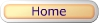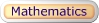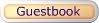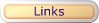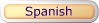### Math Multiplication 3 Multiplication by Factors (Vertical)

This printable basic math multiplication by factors worksheet generator will provides plenty of math practice, boost test scores and prepare the child/children to meet the Grades 3-5 curriculum standards. This math worksheet maker will create a vertical math multiplication, 1 digit by 1 digit (1x1) equations with handwriting lines.

See sample math worksheets below:

 2 x 3 6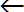factorfactorproduct

1. Select the number of math problems per page. (Default: 1 - 20)
2. Select Type of Math Problems (Default: factors to nine)
3. Click on submit to make your personalized vertical math multipication worksheet.
4. Option: Page back and click Submit to create a different set of math problems.

Math Multiplication 3
Multiplication Worksheet Maker (Vertical)

 Enter the Number of Math Problems per page: Select a range 1 - 5 1 - 10 1 - 15 1 - 20 Default: 1 - 20 Type of Math Problems: Select a Type of Math factor of zero (0) factor of one (1) factor of two (2) factor of three (3) factor of four (4) factor of five (5) factor of six (6) factor of seven (7) factor of eight (8) factor of nine (9) factor of ten (10) factor of eleven (11) factor of twelve (12) factors to one factors to two factors to three factors to four factors to five factors to six factors to seven factors to eight factors to nine factors to ten factors to eleven factors to twelve Default: factors to nineBasic Handwriting for Kids Free for Non-Profit Use Ms. Readman, Rockford, IL Revised: